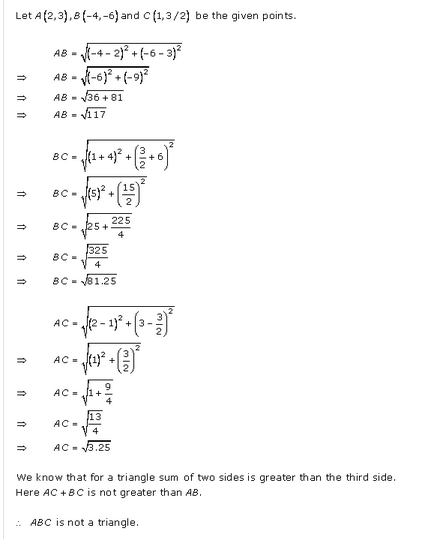## RD Sharma Class 10 Solutions Chapter 14 Coordinate Geometry Ex 14.2

RD Sharma Class 10 Solutions Chapter 14 Coordinate Geometry Ex 14.2 Q1RD Sharma Class 10 Solutions Chapter 14 Ex14.2 Q2Chapter 14 Coordinate Geometry Ex14.2 Q3RD Sharma Class 10 Solutions  Coordinate Geometry Ex14.2 Q4RD Sharma Class 10 Solutions Chapter 14 Coordinate Geometry Ex14.2 Q56.7.8.9.10.11.12.13.14.15.Coordinate Geometry Ex14.2 Q16Coordinate Geometry Ex14.2 Q17Coordinate Geometry Ex14.2 Q18Coordinate Geometry Ex14.2 Q19Coordinate Geometry Ex14.2 Q20Coordinate Geometry Ex14.2 Q20Coordinate Geometry Ex14.2 Q21Coordinate Geometry Ex14.2 Q2223.24.25.26.27.28.29.Ex14.2 Q30Ex14.2 Q31Ex14.2 Q32Ex14.2 Q33Ex14.2 Q34Ex14.2 Q35CBSE RD Sharma Class 10 solutions Ex14.2 Q36CBSE RD Sharma Class 10 solutions Ex14.2 Q37CBSE RD Sharma Class 10 solutions Ex14.2 Q38CBSE RD Sharma Class 10 solutions Ex14.2 Q39CBSE RD Sharma Class 10 solutions Ex14.2 Q40CBSE RD Sharma Class 10 solutions Ex14.2 Q4142.43.RD Sharma Ex 14.2 Q44More Resources For Class 10